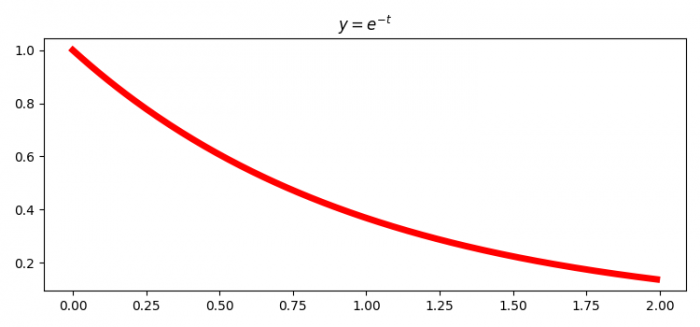# How to close a Python figure by keyboard input using Matplotlib?

MatplotlibPythonData Visualization

To close a Python figure by a keyboard input, we can use plt.pause() method, an input, and close() method.

## Steps

• Set the figure size and adjust the padding between and around the subplots.
• Create random t and y data points using numpy.
• Create a new figure or activate an existing figure using figure() method.
• Plot t and y data points using plot() method.
• Set the title of the plot.
• Redraw the current figure using draw() method.
• Run a true loop to pause the current figure.
• Take input from the user to go to the next statement.
• Use close() method to close the figure.

## Example

import numpy as np
from matplotlib import pyplot as plt
plt.rcParams["figure.figsize"] = [7.50, 3.50]
plt.rcParams["figure.autolayout"] = True
t = np.arange(0.0, 2.0, 0.01)
y = np.exp(-t)
fig = plt.figure()
plt.plot(t, y, lw=5, color='red')
plt.title("$y=e^{-t}$")
plt.draw()
plt.pause(1)
input("<Hit Enter>")
plt.close(fig)

## Output